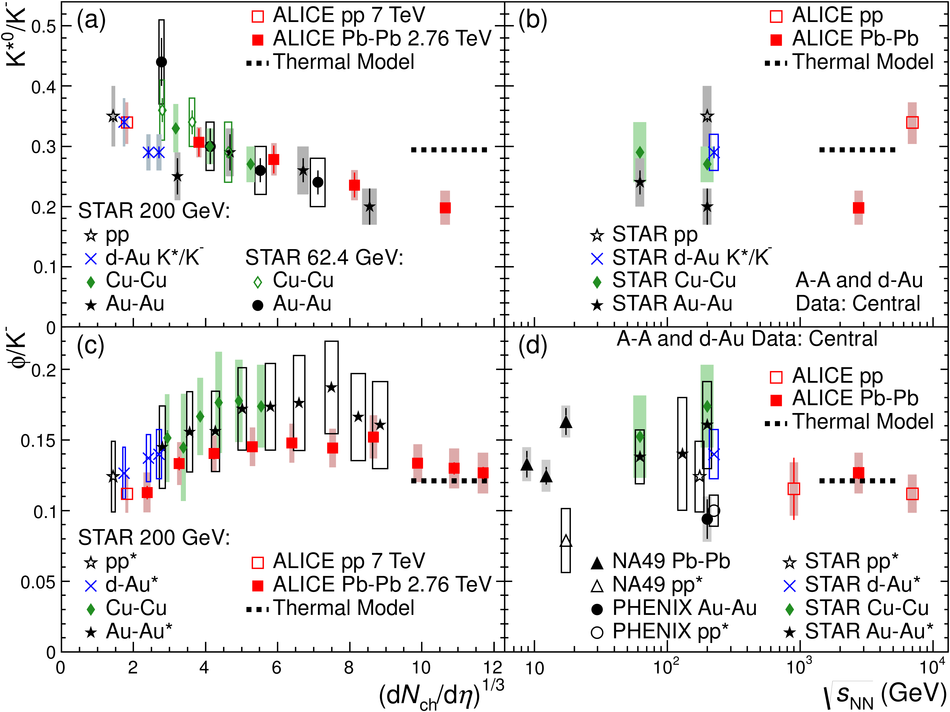# Figure 7

 Particle ratios $\rm K^{*0}/K^{-}$ [panels (a) and (b)] and $\Phi/K^{-}$ [panels (c) and (d)] in pp, d-Au, and A-A collisions. In panels (a) and (c) these ratios are presented for different centrality intervals as a function of $({\rm d} N_{\rm ch}/\rm d\eta)^{1/3}$. The values of ${\rm d} N_{\rm ch}/\rm d\eta$ were measured at mid-rapidity. In panels (b) and (d), these ratios are presented for pp, central d-Au, and central A-A collisions as a function of $\snn$. The values given by a grand-canonical thermal model with a chemical freeze-out temperature of 156 MeV are also shown. For quantities marked *", boxes represent the total uncertainty (separate uncertainties are not reported). Otherwise, bars represent the statistical uncertainties and boxes represent the systematic uncertainties (including centrality-uncorrelated and centrality-correlated components). For the d-Au data in panels (a) and (b), the numerator yield is derived from a combination of the charged and neutral $\mathrm{K}^{*}(892)$ states. In panel (c), the two most central $\Phi/K^{-}$ points for Au-Au collisions are for overlapping centrality intervals (0-5\% and 0-10\%). The following points have been shifted horizontally for visibility: the lowest-multiplicity d-Au points in panels (a) and (c), the d-Au points in panels (b) and (d), and the pp data points for $\snn$ = 200 GeV in panel (d).